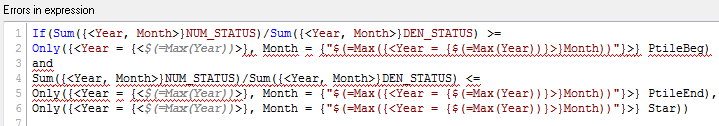# QlikView App Dev

Discussion Board for collaboration related to QlikView App Development.

Announcements
Join this live chat April 6, 10AM EST - QlikView to Qlik Sense REGISTER
cancel
Showing results for
Search instead for
Did you mean:Creator

## Set Analysis not pulling desired field

Hi,

I am currently using the following expression to pull a 'Rate' for an entire year regardless of selections and it is working: Sum({<Year, Month>}NUM_STATUS)/Sum({<Year, Month>}DEN_STATUS) . What I am wanting to do now is assign a 'Star' value based on where that rate falls on the threshold table (between PtileBeg and PtileEnd). However, I need the formula to point to the max Year and Month's thresholds as they vary each month.

This is what I am trying to use but it isn't working:

if(Sum({<Year, Month>}NUM_STATUS)/Sum({<Year, Month>}DEN_STATUS)>= only({<MaxYear,MaxMonth>}PtileBeg) and
Sum({<Year, Month>}NUM_STATUS)/Sum({<Year, Month>}DEN_STATUS)<= only({<MaxYear,MaxMonth>}PtileEnd),only({<MaxYear,MaxMonth>}Star))

Any help is much appreciated.

1 Solution

Accepted SolutionsMVP

Try this

Max({<Year = {\$(=Max({1} Year))}, MonthNum = {"\$(=Max({1<Year = {\$(=Max({1}Year))}>}MonthNum))"}>} PtileBeg)

25 RepliesMVP

May be this

If(Sum({<Year, Month>}NUM_STATUS)/Sum({<Year, Month>}DEN_STATUS) >=

Only({<Year = {<\$(=Max(Year))>}, Month = {"\$(=Max({<Year = {\$(=Max(Year))}>}Month))"}>} PtileBeg)

and
Sum({<Year, Month>}NUM_STATUS)/Sum({<Year, Month>}DEN_STATUS) <=

Only({<Year = {<\$(=Max(Year))>}, Month = {"\$(=Max({<Year = {\$(=Max(Year))}>}Month))"}>} PtileEnd),

Only({<Year = {<\$(=Max(Year))>}, Month = {"\$(=Max({<Year = {\$(=Max(Year))}>}Month))"}>} Star))Creator
Author

Thank you, but I am getting errors and am not sure what may be wrong.Specialist II

I don't know if this is helpful but it might help you get rid of some of the complexity in your expression.

vlookup function in QlikView | Qlik CommunityMVP

My bad, try this

If(Sum({<Year, Month>}NUM_STATUS)/Sum({<Year, Month>}DEN_STATUS) >=

Only({<Year = {\$(=Max(Year))}, Month = {"\$(=Max({<Year = {\$(=Max(Year))}>}Month))"}>} PtileBeg)

and
Sum({<Year, Month>}NUM_STATUS)/Sum({<Year, Month>}DEN_STATUS) <=

Only({<Year = {\$(=Max(Year))}, Month = {"\$(=Max({<Year = {\$(=Max(Year))}>}Month))"}>} PtileEnd),

Only({<Year = {\$(=Max(Year))}, Month = {"\$(=Max({<Year = {\$(=Max(Year))}>}Month))"}>} Star))Creator
Author

I was hoping this would work but it didn't. I think the issue is because of trying to point to the max month/year Ptile. When I just try to show that value it isn't working either. For example, I'm putting this in a text box just to see the value and am getting an error: Only({<Year = {\$(=Max(Year))}, Month = {"\$(=Max({<Year = {\$(=Max(Year))}>}Month))"}>} PtileBeg)MVP

What error message do you see?Creator
Author

Actually it's just null '-', not an error.MVP

Try with Max

Max({<Year = {\$(=Max(Year))}, Month = {"\$(=Max({<Year = {\$(=Max(Year))}>}Month))"}>} PtileBeg)Creator
Author

Same thingI also tried this and not getting any result: =max({<Month = {\$(=Max(Month))}>} PtileBeg)